Courses

# First Law Of Thermodynamics MSQ

## 10 Questions MCQ Test Basic Physics for IIT JAM | First Law Of Thermodynamics MSQ

Description
This mock test of First Law Of Thermodynamics MSQ for Physics helps you for every Physics entrance exam. This contains 10 Multiple Choice Questions for Physics First Law Of Thermodynamics MSQ (mcq) to study with solutions a complete question bank. The solved questions answers in this First Law Of Thermodynamics MSQ quiz give you a good mix of easy questions and tough questions. Physics students definitely take this First Law Of Thermodynamics MSQ exercise for a better result in the exam. You can find other First Law Of Thermodynamics MSQ extra questions, long questions & short questions for Physics on EduRev as well by searching above.
*Multiple options can be correct
QUESTION: 1

### In case of isothermal expansion, which of the following are not forbidden? Select one or more:

Solution:

Let psurrdV  work done by an ideal gas in the three types of expansion.
psurr is a pressure surrounding
Since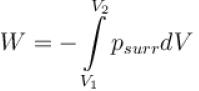The maximum work is done when the gas pressure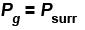For reversible expansion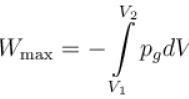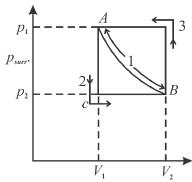Path 2 corresponds to a permitted or irreversible expansion, in this case, work done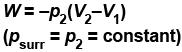In a forbidden expansion i.e. one against an external pressure greater than the gas pressure, the work done by the gas would have to be grater than the maximum work, such an expansion is never observed.
∴ In case of isothermal expansion
Permitted process :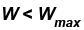Reversible process :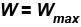Forbidden process :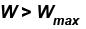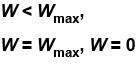*Multiple options can be correct
QUESTION: 2

### A thermos bottle containing coffee is vigorously shaken and thereby the temperature of coffee rises. Regard the coffee as the system. Select one or more:

Solution:

The heat has not been transferred to coffee which is thermally insulated by shaking, work has been done on coffee (system) against the viscous forces in it.
According to first law,
ΔU = Q – W
Here Q = 0
And W is negative as work is done on the system so that
ΔU = 0 – (–W)
i.e. ΔU is positive. The internal energy of system (coffee) increases.
Q = 0, W = -ve, ΔU = +ve
The correct answers are: Q = 0, W = –ve, ΔU = +ve

*Multiple options can be correct
QUESTION: 3

### Which type of ideal gas will have largest value of CP – CV? Select one or more:

Solution:

For an ideal gas,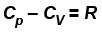Whatever be the number of atoms each gas molecule has
The correct answers are: Monatomic, Diatomic, Polyatomic

*Multiple options can be correct
QUESTION: 4

Which among the following statements are correct?
Select one or more:

Solution:

Energy changes with mass of the body, therefore, it is an extensive property.
Specific energy is the energy of the system per unit mass of the system, therefore, it will become intensive property. Heat capacity is product of specific heat and mass of the body. It depends on mass of the system,
∴ heat capacity is an extensive property. Internal energy of the system is independent of the path followed by system. It has fixed value along the path
∴ energy is the point function
The correct answers are: Energy is an extensive property, Energy is a point function, Heat capacity is an extensive property

*Multiple options can be correct
QUESTION: 5

Which of the following processes must violate the first law of thermodynamics?
Select one or more:

Solution:

From first law of thermodynamics,
ΔU = Q + W
in option (b), W > 0 & Q > 0
⇒ ΔU has to be positive but ΔU < 0
The correct answer is: W > 0, Q < 0, ΔU < 0

*Multiple options can be correct
QUESTION: 6

1 mole of ideal monoatomic gas at 27ºC expands under adiabatic conditions at a pressure of 1.5 atm from a volume of 4dm3 to 16dm3.
Select one or more:

Solution:

Since processes is adiabatic q = 0.
As the gas expands against constant external processes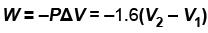= –1.5(16–4) = –18atm-dm3
ΔU = Q + W = 0 + (–18) = –18atm-dm3
The correct answers are:  W = –18atm – dm3 , ΔU = –18atm – dm3

*Multiple options can be correct
QUESTION: 7

In an isothermal expansion of an ideal gas,
Select one or more:

Solution:

For one mole of an ideal gas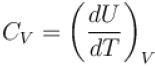⇒ dU = CVdT
For isothermal process, T is constant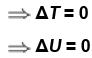Also ΔH = ΔU + Δ(pV)
pV = RT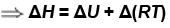= ΔU + RΔT
ΔH = 0.
The correct answers are: ΔU = 0, ΔH = 0

*Multiple options can be correct
QUESTION: 8

Which of the following are path function?
Select one or more:

Solution:

Heat transfer as well as work transfer between the system and surrounding depends upon the path by which the process is occurred. Therefore heat energy and work energy are path function. The change in internal energy ΔE remains constant, no matter which path is followed by a system to undergo a change of a certain state. Thus, internal energy is a point function or state function.
The correct answers are: Heat Energy, Work

*Multiple options can be correct
QUESTION: 9

A resistor immersed in running water carries an electric current. Consider the resistor as the system.
Select one or more:

Solution:

There is no flow of heat into the resistor and work is done on the resistor (system).
According to first law as applied to the system, we write,
ΔU = Q – W
For resistor Q = 0
W = –ve. Thus
ΔU = 0 – (–W) = W
∴ Q = 0; W = –ve, ΔU = +ve
The correct answers are: Q = 0, W = –ve, ΔU = +ve

*Multiple options can be correct
QUESTION: 10

Consider that 214 J of work done on a system, and 293 J of heat are extracted from the system. Then
Select one or more:

Solution:

Since work is done on the system, therefore, algebraic sign of work done will be positive and magnitude of work done is 214J.
Since heat is extracted from the system,
∴ Q = –293 J
Now ΔU = Q + W
= –293 J + 214 J
ΔU = –79 J
The correct answers are: Q = –293 J, ΔU = –79 J